# Lakhmir Singh solutions for Class 10 Physics (Science) chapter 1 - Electricity [Latest edition]

#### Chapters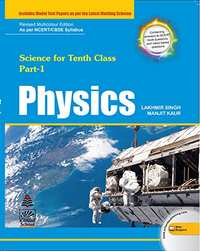## Solutions for Chapter 1: Electricity

Below listed, you can find solutions for Chapter 1 of CBSE Lakhmir Singh for Class 10 Physics (Science).

Exercise 1Exercise 2Exercise 3Exercise 4Exercise 5Exercise 6Exercise 7Exercise 8
Exercise 1

### Lakhmir Singh solutions for Class 10 Physics (Science) Chapter 1 Electricity Exercise 1

Exercise 1 | Q 1

By what other name is the unit joule/coulomb called?

Exercise 1 | Q 2

Which of the following statements correctly defines a volt?
(a) a volt is a joule per ampere.
(b) a volt is a joule per coulomb.

Exercise 1 | Q 3.1

What do the letters p.d. stand for?

Exercise 1 | Q 3.2

Which device is used to measure p.d?

Exercise 1 | Q 4

What is meant by saying that the electric potential at a point is 1 volt?

Exercise 1 | Q 5

How much work is done when one coulomb charge moves against a potential difference of 1 volt?

Exercise 1 | Q 6

What is the SI unit of potential difference?

Exercise 1 | Q 7

How much work is done in moving a charge of 2 C across two points having a potential difference of 12 V?

Exercise 1 | Q 8

What is the unit of electric charge?

Exercise 1 | Q 9

Define one coulomb charge.

Exercise 1 | Q 10.1

Potential difference is measured in _________ by using a ___________ placed in ___________ across a component.

Exercise 1 | Q 10.2

Fill in the following blanks with suitable words:

Copper is a good .......... Plastic is an ..........

Exercise 1 | Q 11

What is meant by conductors and insulators? Give two examples of conductors and two of insulators.

Exercise 1 | Q 12

Which of the following are conductors and which are insulators?

Sulphur, Silver, Copper, Cotton, Aluminium, Air, Nichrome, Graphite, Paper, porcelain, Mercury, Mica, Bakelite, Polythene, Manganin.

Exercise 1 | Q 13

What do you understand by the term "electric potential"? (or potential) at a point? What is the unit of electric potential?

Exercise 1 | Q 14.1

State the relation between potential difference, work done and charge moved.

Exercise 1 | Q 14.2

Calculate the work done in moving a charge of 4 coulombs from a point at 220 volts to another point at 230 volts.

Exercise 1 | Q 15.1

Name a device that help to measure the potential difference across a conductor.

Exercise 1 | Q 15.2

How much energy is transferred by a 12 V Power supply to each coulomb of charge which it moves around a circuit?

Exercise 1 | Q 16.1

What do you understand by the term "potential difference"?

Exercise 1 | Q 16.2

What is meant by saying that the potential difference between two points is 1 V?

Exercise 1 | Q 16.3

What is the potential difference between the terminals of a battery if 250 joules of work is required to transfer 20 coulombs of charge from one terminal of battery to the other?

Exercise 1 | Q 16.4

How is a voltmeter connected in the circuit to measure the potential different between two points. Explain with the help of a diagram.

Exercise 1 | Q 16.4

What is a voltmeter?

Exercise 1 | Q 16.5

State whether a voltmeter has a high resistance of a low resistance. Give reason for your answer.

Exercise 1 | Q 17

The work done in moving a unit charge across two points in an electric circuit is a measure of:

(a) current
(b) potential difference
(c) resistance
(d) power

Exercise 1 | Q 17.3

<p> Define the unit of electric current (or Define ampere).

Exercise 1 | Q 18

The device used for measuring potential difference is known as:

• potentiometer

• ammeter

• galvanometer

• voltmeter

Exercise 1 | Q 19

Which of the following units could be used to measure electric charge?

(a) ampere
(b) joule
(c) volt
(d) coulomb

Exercise 1 | Q 20

The unit for measuring potential difference is:

• watt

• watt

• ohm

• ohm

• volt

• volt

• kWh

• kWh

Exercise 1 | Q 21

One coulomb charge is equivalent to the charge contained in:

(a) 2.6 × 1019 electrons
(b) 6.2 × 1019 electrons
(c) 2.65 × 1018 electrons
(d) 6.25 × 1018 electrons

Exercise 1 | Q 22.1

Three 2 V cells are connected in series and used as a battery in a circuit.

What is the p.d. at the terminals of the battery?

Exercise 1 | Q 22.2

Three 2 V cells are connected in series and used as a battery in a circuit.

How many joules of electrical energy does 1 C gain on passing through (i) one cell (ii) all three cells?

Exercise 1 | Q 23

The atoms of copper contain electrons and the atoms of rubber also contain electrons. Then why does copper conduct electricity but rubber does not conduct electricity?

Exercise 1 | Q 27

The other name of potential difference is:

• Amperage

• Wattage

• Voltage

• Potential energy

Exercise 1 | Q 1

An electric iron is connected to the mains power supply of 220 V. When the electric iron is adjusted at 'minimum heating' it consumes a power of 360 W but at 'maximum heating' it takes a power of 840 W. Calculate the current and resistance in each case.

Exercise 2

### Lakhmir Singh solutions for Class 10 Physics (Science) Chapter 1 Electricity Exercise 2

Exercise 2 | Q 1

By what name is the physical quantity coulomb/second called?

Exercise 2 | Q 2

What is the Flow of Charge Called?

Exercise 2 | Q 3

What actually travels through the wires when you switch on a light?

Exercise 2 | Q 4

Which particles constitute the electric current in a metallic conductor?

Exercise 2 | Q 5.1

In which direction does conventional current flow around a circuit?

Exercise 2 | Q 5.2

In which direction do electrons flow?

Exercise 2 | Q 6

Which of the following equation shows the correct relationship between electrical unit?

1A = 1C/s or 1c= 1A/s

Exercise 2 | Q 7

What is the unit of electric current?

Exercise 2 | Q 8.1

How many milliamperes as there in 1 ampere?

Exercise 2 | Q 8.2

How many microamperes are there in 1 ampere?

Exercise 2 | Q 9

Which of the two is connected in series : ammeter or voltmeter?

Exercise 2 | Q 10

Compare how an ammeter and a voltmeter are connected in a circuit.

Exercise 2 | Q 11.1

What do the following symbols mean in circuit diagrams?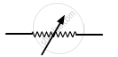Exercise 2 | Q 11.2

What do the following symbols mean in circuit diagrams?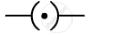Exercise 2 | Q 12

If 20 C of charge pass a point in a circuit in 1 s, what current is flowing?

Exercise 2 | Q 13

A current of 4 A flows around a circuit for 10 s. How much charge flows past a point in the circuit in this time?

Exercise 2 | Q 14

What is the current in a circuit if the charge passing each point is 20 C in 40 s?

Exercise 2 | Q 15.1

Fill in the folloing blank with suitable words:

A current is a flow of ............... .For this to happen there must be a ............... circuit.

Exercise 2 | Q 15.2

Fill in the folloing blank with suitable words:

Current is measured in ............... using an ............... placed in ............... in a circuit.

Exercise 2 | Q 16

Name a device which helps to maintain potential difference across a conductor (say, a bulb).

Exercise 2 | Q 16.2

If a potential difference of 10 V causes a current of 2 A to flow for 1 minute, how much energy is transferred?

Exercise 2 | Q 17.1

What is an electric current?

Exercise 2 | Q 17.2

What Makes an Electric Current Flow in a Wire?

Exercise 2 | Q 18

What is an Ammeter? How is It Connected in a Circuit? Draw a Diagram to Illustrate Your Answer.

Exercise 2 | Q 19.1

Write down the formula which relates electric charge, time and electric current

Exercise 2 | Q 19.2

A Radio Set Draws a Current of 0.36 a for 15 Minutes. Calculate the Amount of Electric Charge that Flows Through the Circuit.

Exercise 2 | Q 20.1

Why should the resistance of:

an ammeter be very small?

Exercise 2 | Q 20.2

Why should the resistance of:

a voltmeter be very large?

Exercise 2 | Q 21.1

Draw circuit symbols for

fixed resistance

Exercise 2 | Q 21.2

Draw circuit symbols for variable resistance

Exercise 2 | Q 21.3

Draw circuit symbols for

a cell

Exercise 2 | Q 21.4

Draw circuit symbols for

a battery of three cells

Exercise 2 | Q 21.5

Draw circuit symbols for

an open switch

Exercise 2 | Q 21.6

Draw circuit symbols for

A closed switch

Exercise 2 | Q 22.1

What is a circuit diagram

Exercise 2 | Q 22.2

Draw the labelled diagram of an electric circuit comprising of a cell, a resistance, an ammeter, a voltmeter and a closed switch (or closed plug key). Which of the two has a large resistance : an ammeter or a voltmeter?

Exercise 2 | Q 23

If the charge on an electron is 1.6 × 1019 coulombs, how many electrons should pass through a conductor in 1 second to constitute 1 ampere current?

Exercise 2 | Q 24.1

The p.d. across a lamp is 12 V. How many joules of electrical energy are changed into heat and light when:

a charge of 1 C passes through it?

Exercise 2 | Q 24.2

The p.d. across a lamp is 12 V. How many joules of electrical energy are changed into heat and light when:

a charge of 5 C passes through it?

Exercise 2 | Q 24.3

The p.d. across a lamp is 12 V. How many joules of electrical energy are changed into heat and light when: a current of 2 A flows through it for 10 s?

Exercise 2 | Q 25.1

In 10 s, charge of 25 C leaves a battery, and 200 J of energy are delivered to an outside circuit a result.

What is the p.d. across the battery?

Exercise 2 | Q 25.2

In 10 s, charge of 25 C leaves a battery, and 200 J of energy are delivered to an outside circuit a result.

What current flows from the battery?

Exercise 2 | Q 26.1

Define electric current. What is the SI unit of electric current.

Exercise 2 | Q 26.2

One coulomb of charge flows through any cross-section of a conductor in 1 second. What is the current flowing through the conductor?

Exercise 2 | Q 26.3

Which instrument is used to measure electric current? How should it be connected in a circuit?

Exercise 2 | Q 26.4

What is the conventional direction of the flow of electric current? How does it differ from the direction of flow electrons?

Exercise 2 | Q 26.5

A flash of lighting carries 10 C of charge which flows for 0.01 s. What is the current? If the voltage is 10 MV, what is the energy?

Exercise 2 | Q 28

Which statement/statement is/are correct?
1. An ammeter is connected in series in a circuit and a voltmeter is connected in parallel.
2. An ammeter has a high resistance.
3. A voltmeter has a low resistance.

(a) 1, 2, 3
(b) 1, 2
(c) 2, 3
(d) 1

Exercise 2 | Q 29

Which unit could be used to measure current?

(a) Watt
(b) Coulomb
(c) Volt
(d) Ampere

Exercise 2 | Q 30

If the current through a floodlamp is 5 A, what charge passed in 10 seconds?

(a) 0.5 C
(b) 2 C
(c) 5 C
(d) 50 C

Exercise 2 | Q 31

If the amount of electric charge passing through a conductor in 10 minutes is 300 C, the current flowing is:

(a) 30 A
(b) 0.3 A
(c) 0.5 A
(d) 5 A

Exercise 2 | Q 32

A student made an electric circuit shown here to measure the current through two lamps.

Are the lamps in series or parallel?

(a) Are the lamps in series or parallel?
(b) The student has made a mistake in this circuit. What is the mistake?
(c) Draw a circuit diagram to show the correct way to connect the circuit. Use the proper circuit symbols in your diagram.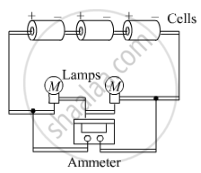Exercise 2 | Q 33

Draw a circuit diagram to show how 3 bulbs can be lit from a battery so that 2 bulbs are controlled by the same switch the third bulb has its own switch.

Exercise 2 | Q 34.1

An electric heater is connected to the 230 V mains supply. A current of 8 A flows through the heater.

(a) How much charge flows around the circuit each second?

Exercise 2 | Q 34.2

An electric heater is connected to the 230 V mains supply. A current of 8 A flows through the heater.

(b) How much energy is transferred to the heater each second?

Exercise 2 | Q 35

How many electrons are flowing per second past a point in a circuit in which there is a current of 5 amp?

Exercise 3

### Lakhmir Singh solutions for Class 10 Physics (Science) Chapter 1 Electricity Exercise 3

Exercise 3 | Q 1

Name the law which relates the current in a conductor to the potential difference across its ends.

Exercise 3 | Q 2

Name the unit of electrical resistance and give its symbol.

Exercise 3 | Q 3

Name the physical quantity whose unit is "ohm".

Exercise 3 | Q 4

What is the general name of the substances having infinitely high electrical resistance?

Exercise 3 | Q 5

Keeping the resistance constant, the potential difference applied across the ends of a component is halved. By how much does the current change?

Exercise 3 | Q 6

State the factors on which the strength of electric current flowing in a given conductor depends.

Exercise 3 | Q 7

Which has less electrical resistance : a thin wire or a thick wire (of the same length and same material)?

Exercise 3 | Q 8

Keeping the potential difference constant, the resistance of a circuit is halved. By how much does the current change?

Exercise 3 | Q 9

A potential difference of 20 volts is applied across the ends of a resistance of 5 ohms. What current will flow in the resistance?

Exercise 3 | Q 10

A resistance of 20 ohms has a current of 2 amperes flowing in it. What potential difference is there between its ends?

Exercise 3 | Q 10

Why are the coils of electric irons and electric toasters made of an alloy rather than a pure metal?

Exercise 3 | Q 11

A current of 5 amperes flows through a wire whose ends are at a potential difference of 3 volts. Calculate the resistance of the wire.

Exercise 3 | Q 12

Ohm's law state a relation between potential difference and ___________.

Exercise 3 | Q 13

Distinguish between good conductors, resistors and insulators. Name two good conductors, two resistance and two insulators.

Exercise 3 | Q 14

Classify the following into good conductors, resistors and insulators:

Rubber, Mercury, Nichrome, Polythene, Aluminium, Wood, Manganin, Bakelite, Iron, Paper, Thermocol, Metal coin

Exercise 3 | Q 15

What is Ohm's law? Explain how it is used to define the unit of resistance.

Exercise 3 | Q 16.1

What is meant by the "resistance of a conductor" ? Write the relation between resistance, potential difference and current.

Exercise 3 | Q 16.2

When a 12 V battery is connected across an unknown resistor, there is a current of 2.5 mA in the circuit. Calculate the value of the resistance of the resistor.

Exercise 3 | Q 17.1

Define the unit of resistance (or Define the unit "ohm").

Exercise 3 | Q 17.2

What happens to the resistance as the conductor is made thinner?

Exercise 3 | Q 17.3

Keeping the potential difference constant, the resistance of a circuit is doubled. By how much does the current change?

Exercise 3 | Q 18.1

Why do electricians wear rubber hand gloves while working with electricity?

Exercise 3 | Q 18.2

What p.d. is needed to send a current of 6 A through an electrical appliance having a resistance of 40 Ω?

Exercise 3 | Q 19.1

An electric circuit consisting of a 0.5 m long nichrome wire XY, an ammeter, a voltmeter, four cells of 1.5 V each and a plug key was set up.

(i) Draw a diagram of this electric circuit to study the relation between the potential difference maintained between the points 'X' and 'Y' and the electric current flowing through XY.

Exercise 3 | Q 19.2

An electric circuit consisting of a 0.5 m long nichrome wire XY, an ammeter, a voltmeter, four cells of 1.5 V each and a plug key was set up.

Following graph was plotted between V and I values: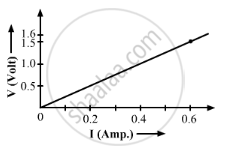What would be the values of V/I rations when the potential difference is 0.8 V, 1.2 V and 1.6 V respectively?
What conclusion do you draw from these values?

What is the resistance of the wire?

Exercise 3 | Q 20.1

What is the ratio of potential difference and current known as?

Exercise 3 | Q 20.2

The values of potential difference V applies across a resistor and the corresponding values of current I flowing in the resistor are given below:

 Potential differences, V (in volts) : 2.5 5 10 15 20 25 Current, I (in amperes) : 0.1 0.2 0.4 0.6 0.8 1
Exercise 3 | Q 20.3

Name the law which is illustrated by the above VI graph.

Exercise 3 | Q 20.4

Write down the formula which states the relation between potential difference, current, and resistance.

Exercise 3 | Q 21

The p.d. across a 3 Ω resistor is 6 V. The current flowing in the resistor will be:

(a) 1/2A
(b) 1 A
(c) 2 A
(d) 6 A

Exercise 3 | Q 22

A car headlight bulb working on a 12 V car battery draws a current of 0.5 A. The resistance of the light bulb is:

(a) 0.5 Ω
(b) 6 Ω
(c) 12 Ω
(d) 24 Ω

Exercise 3 | Q 23

An electrical appliance has a resistance of 25 Ω. When this electrical appliance is connected to a 230 V supply line, the current passing through it will be:

(a) 0.92 A
(b) 2.9 A
(c) 9.2 A
(d) 92 A

Exercise 3 | Q 24

When a 4 Ω resistor is connected across the terminals of a 12 V battery, the number of coulombs passing through the resistor per second is:

(a) 0.3
(b) 3
(c) 4
(d) 12

Exercise 3 | Q 25

Ohm's law gives a relationship between:

(a) current and resistance
(b) resistance and potential difference
(c) potential difference and electric charge
(d) current and potential difference

Exercise 3 | Q 26

The unit of electrical resistance is:

(a) ampere
(b) volt
(c) coulomb
(d) ohm

Exercise 3 | Q 27

The substance having infinitely high electrical resistance is called:

(a) conductor
(b) resistor
(c) superconductor
(d) insulator

Exercise 3 | Q 28

Keeping the potential difference constant, the resistance of a circuit is doubled. The current will become:

• double

• half

• one-fourth

• Four times

Exercise 3 | Q 29

Keeping the p.d. constant, the resistance of a circuit is halved. The current will become:

(a) one-fourth
(b) four time
(c) half
(d) double

Exercise 3 | Q 30

An electric room heater draws a current of 2.4 A from the 120 V supply line. What current will this room heater draw when connected to 240 V supply line?

Exercise 3 | Q 31

Name the electrical property of a material whose symbol is "omega".

Exercise 3 | Q 32.1

The graph between V and I for a conductor is a straight line passing through the origin.

Which law is illustrated by such a graph?

Exercise 3 | Q 32.2

The graph between V and I for a conductor is a straight line passing through the origin.

What should remain constant in a statement of this law?

Exercise 3 | Q 33

A p.d. of 10 V is needed to make a current of 0.02 A flow through a wire. Wire p.d. is needed to make a current of 250 mA flow through the same wire?

Exercise 3 | Q 34

A current of 200 mA flows through a 4 kΩ resistor. What is the p.d. across the resistor?

Exercise 3 | Q 205

The potential difference between the terminals of an electric iron is 240 V and the current is 5.0 A. What is the resistance of the electric iron?

Exercise 4

### Lakhmir Singh solutions for Class 10 Physics (Science) Chapter 1 Electricity Exercise 4

Exercise 4 | Q 1

What happens to the resistance as the conductor is made thicker?

Exercise 4 | Q 2

If the length of a wire is doubled by taking more of wire, what happens to its resistance?

Exercise 4 | Q 3

On what factors does the resistance of a conductor depend?

Exercise 4 | Q 4

Name the material which is the best conductor of electricity.

Exercise 4 | Q 5

Which among iron and mercury is a better conductor of electricity?

Exercise 4 | Q 6

Why are copper and aluminium wires usually employed for electricity transmission?

Exercise 4 | Q 7

Name the material which is used for making the heating element of an electric iron.

Exercise 4 | Q 8

What is nichrome? State its one use.

Exercise 4 | Q 9

Give two reasons why nichrome alloy is used for making the heating elements of electrical appliances.

Exercise 4 | Q 11.1

Which has more resistance:

a long piece of nichrome wire or a short one?

Exercise 4 | Q 11.2

Which has more resistance:

a thick piece of nichrome wire or a thin piece?

Exercise 4 | Q 12.1

How does the resistance of a pure metal change if its temperature decreases?

Exercise 4 | Q 12.2

How does the presence of impurities in a metal affect its resistance?

Exercise 4 | Q 13

Fill in the following blank with suitable words:

Resistance is measured in .............. The resistance of a wire increases as the length ..............; as the temperature ..............; and as the cross-sectional area .............. .

Exercise 4 | Q 14.1

What do you understand by the "resistivity" of a substance?

Exercise 4 | Q 14.2

A wire is 1.0 m long, 0.2 mm in diameter and has a resistance of 10 Ω. Calculate the resistivity of its material?

Exercise 4 | Q 15.1

Write down an expression for the resistance of a metallic wire in terms of the resistivity.

Exercise 4 | Q 15.2

What will be the resistance of a metal wire of length 2 metres and area of cross-section 1.55 × 10−6 m2 if the resistivity of the metal be 2.8 × 10−8 Ω m?

Exercise 4 | Q 16.1

Give two examples of substances which are good conductors of electricity. Why do you think they are good conductors of electricity?

Exercise 4 | Q 16.2

Calculate the resistance of a copper wire 1.0 km long and 0.50 mm diameter if the resistivity of copper is 1.7 × 10−8 Ω m.

Exercise 4 | Q 17

Will current flow more easily through a thick wire or a thin wire of the same material, when connected to the same source? Why?

Exercise 4 | Q 18.1

How does the resistance of a conductor depend on:

length of the conductor?

Exercise 4 | Q 18.2

How does the resistance of a conductor depend on:

area of cross-section of the conductor?

Exercise 4 | Q 18.3

How does the resistance of a conductor depend on:

temperature of the conductor?

Exercise 4 | Q 19.2

Calculate the resistance of an aluminium cable of length 10 km and diameter 2.0 mm if the resistivity of aluminium is 27 × 10−8 Ωm.

Exercise 4 | Q 19.3

Give one example to show how the resistance depends on the nature of material of the conductor.

Exercise 4 | Q 20.1

What would be the effect on the resistance of a metal wire of:

increasing its length?

Exercise 4 | Q 20.2

What would be the effect on the resistance of a metal wire of:

increasing its diameter?

Exercise 4 | Q 20.3

What would be the effect on the resistance of a metal wire of:

increasing its temperature?

Exercise 4 | Q 21.1

How does the resistance of a wire vary with its area of cross-section?

Exercise 4 | Q 21.2

How does the resistance of a wire vary with its:

diameter?

Exercise 4 | Q 22.1

How does the resistance of a wire change when:

its length is tripled?

Exercise 4 | Q 22.2

How does the resistance of a wire change when:

its diameter is tripled?

Exercise 4 | Q 22.3

How does the resistance of a wire change when:

its material is changed to one whose resistivity is three times?

Exercise 4 | Q 23

Calculate the area of cross-section of a wire if its length is 1.0 m, its resistance is 23 Ω and the resistivity of the material of the wire is 1.84 × 10−6 Ω m.

Exercise 5

### Lakhmir Singh solutions for Class 10 Physics (Science) Chapter 1 Electricity Exercise 5

Exercise 5 | Q 1

Give the law of combination of resistances in series.

Exercise 5 | Q 2

If five resistances, each of value 0.2 ohm, are connected in series, what will be the resultant resistance?

Exercise 5 | Q 3

Which of the following arrangement, A or B, has the lower combined resistance?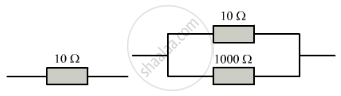Exercise 5 | Q 3

State the law of combination of resistances in parallel.

Exercise 5 | Q 5

How should the two resistances of 2 ohms each be connected so as to produce an equivalent resistance of 1 ohm?

Exercise 5 | Q 6

V = IRTwo resistances X and Y are connected turn by turn : (i) in parallel, and (ii) series. In which case the resultant resistance will be less than either of the individual resistances?R = R1+ R2+ R3

Exercise 5 | Q 7

What possible values of resultant resistance one can get by combining two resistances, one of value 2 ohm and the other 6 ohm?

Exercise 5 | Q 8

Show how you would connect two 4 ohm resistors to produce a combined resistance of (a) 2 ohms (b) 8 ohms.

Exercise 5 | Q 10

A wire that has resistance R is cut into two equal pieces. The two parts are joined in parallel. What is the resistance of the combination?

Exercise 5 | Q 11

Calculate the combined resistance in each case: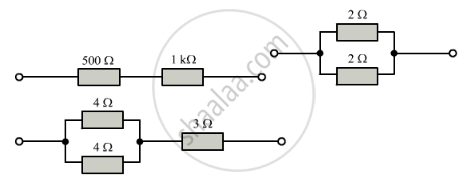Exercise 5 | Q 12

Find the current in each resistor in the circuit shown below: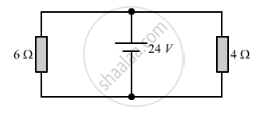Exercise 5 | Q 13

Explain with diagram what is meant by the "series combination" and "parallel combination" of resistances. In which case the resultant resistance is : (i) less, and (ii) more, than either of the individual resistances?

Exercise 5 | Q 14

A battery of 9 V is connected in series with resistors of 0.2 Ω, 0.3 Ω, 0.4 Ω, 0.5 Ω and 0.12 Ω. How much current would flow through the 12 Ω resistor?

Exercise 5 | Q 15

An electric bulb of resistance 20 Ω and a resistance wire of 4 Ω are connected in series with a 6 V battery.
Draw the circuit diagram, and calculate:

(a) total resistance of the circuit.
(b) current through the circuit.
(c) potential difference across the electric bulb.
(d) potential difference across the resistance wire.

Exercise 5 | Q 16

Three resistors are connected as shown in the diagram.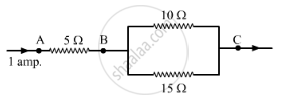Through the resistor 5 ohm, a current of 1 ampere is flowing.

(i) What is the current through the other two resistors?
(ii) What is the p.d. across AB and across AC?
(iii) What is the total resistance

Exercise 5 | Q 17

For the circuit shown in the diagram below: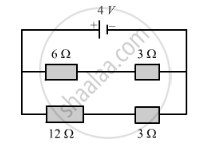What is the value of:
(i) current through 6 Ω resistor?
(ii) potential difference across 12 Ω resistor?

Exercise 5 | Q 18

Two resistors, with resistance 5 Ω and 10 Ω respectively are to be connected to a battery of emf 6 V so as to obtain:

(i) minimum current flowing
(ii) maximum current flowing
(a) How will you connect the resistances in each case?
(b) Calculate the strength of the total current in the circuit in the two cases.

Exercise 5 | Q 19

The circuit diagram given below shows the combination of three resistors R1, R2 and R3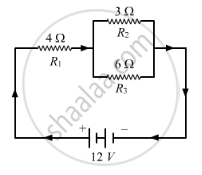Find :
(i) total resistance of the circuit.
(ii) total current flowing in the circuit.
(iii) the potential difference across R1.

Exercise 5 | Q 20

In the circuit diagram given below, the current flowing across 5 ohm resistor is 1 amp. Find the current flowing through the other two resistors.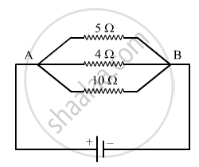Exercise 5 | Q 21

A resistor has a resistance of 176 ohms. How many of these resistors should be connected in parallel so that their combination draws a current of 5 amperes from a 220 volt supply line?

Exercise 5 | Q 22

An electric heater which is connected to a 220 V supply line has two resistance coils A and B of 24 Ω resistance each. These coils can be used separately (one at a time), in series or in parallel. Calculate the current drawn when:

(a) only one coil A is used.
(b) coils and B are used in series.
(c) coils and B are uses in parallel.

Exercise 5 | Q 23

In the circuit diagram given below five resistances of 10 Ω, 40 Ω, 30 Ω, 20 Ω, and 60 Ω are connected as shown to a 12 V battery.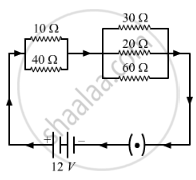Calculate:
(a) total resistance in the circuit.

(b) total current flowing in the circuit.

Exercise 5 | Q 24

In the circuit diagram  given below, three resistors R1, R2, and R3 of 5 Ω, 10 Ω and 30 Ω, respectively are connected as shown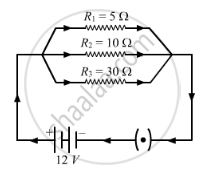Calculate:
(a) current through each resistor.

(b) total current in the circuit.

(c) total resistance in the circuit.

Exercise 5 | Q 25

A p.d. of 4 V is applied to two resistors of 6 Ω and 2 Ω connected in series. Calculate:

(a) the combined resistance
(b) the current flowing
(c) the p.d. across the 6 Ω resistor

Exercise 5 | Q 26

A p.d. of 6 V is applied to two resistors of 3 Ω and 6 Ω connected in parallel. Calculate:

(a) the combined resistance
(b) the current flowing in the main circuit
(c) the current flowing in the 3 Ω resistor.

Exercise 5 | Q 27

In the circuit shown below, the voltmeter reads 10 V.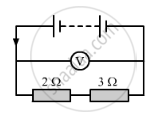(a) What is the combined resistance?
(b) What current flows?
(c) What is the p.d. across 2 Ω resistor?
(d) What is the p.d. across 3 Ω resistor?

Exercise 5 | Q 28

In the circuit given below: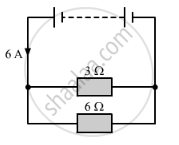(a) What is the combined resistance?
(b) What is the p.d. across the combined resistor?
(c) What is the p.d. across the 3 Ω resistor?
(d) What is the current in the 3 Ω resistor?
(e) What is the current in the 6 Ω resistor?

Exercise 5 | Q 29

A 5 V battery is connected to two 20 Ω resistors which are joined together in series.

(a) Draw a circuit diagram to represent this. Add an arrow to indicate the direction of conventional current flow in the circuit.
(b) What is the effective resistance of the two resistors?
(c) Calculate the current that flows from the battery?
(d) What is the p.d. across each resistor?

Exercise 5 | Q 30

The figure given below shows an electric circuit in which current flows from a 6 V battery through two resistors.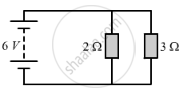(a) Are the resistors connected in series with each other or in parallel?
(b) For each resistors, state the p.d. across it.
(c) The current flowing from the battery is shared between the two resistors. Which resistor will have bigger share of the current?
(d) Calculate the effective resistance of the tow resistors.
(e) Calculate the current that flows form the battery.

Exercise 5 | Q 31

A 4 Ω coil and a 2 Ω coil are connected in parallel. What is their combined resistance? A total current of 3 A passes through the coils. What current passes through the 2 Ω coil?

Exercise 5 | Q 34

(a) With the help of a circuit diagram, obtain the relation for the equivalent resistance of two resistances connected in parallel.
(b) In the circuit diagram shown below, find:

(i) Total resistance.
(ii) Current shown by the ammeter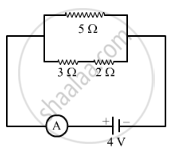Exercise 5 | Q 35.1

Explain with the help of a labelled circuit diagram, how you will find the resistance of a combination of three resistors of resistances R1, R2 and R3 joined in parallel.

Exercise 5 | Q 35.2

In the diagram shown below, the cell and the ammeter both have negligible resistance. The resistor are identical.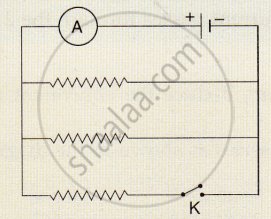Exercise 5 | Q 36

The figure given below shows three resistors?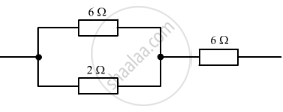Their combined resistance is:

(a) 1 5/7Ω

(b) 14 Ω

(c) 6 2/3 Ω

(d) 7 1/2 Ω

Exercise 5 | Q 37

If two resistors of 25 Ω and 15 Ω are joined together in series and then placed in parallel with a 40 Ω resistor, the effective resistance of the combination is :

(a) 0.1 Ω
(b) 10 Ω
(c) 20 Ω
(d)40 Ω

Exercise 5 | Q 38

The diagram below shows part of a circuit: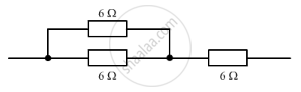If this arrangement of three resistors was to be replaced by a single resistor, its resistance should be:

(a) 9 Ω
(b) 4 Ω
(c) 6 Ω
(d) 18 Ω

Exercise 5 | Q 39

In the circuit shown below: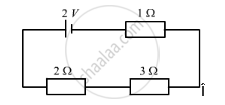The potential difference across the 3 Ω resistor is:

• 1/9V

• 1/2 V

• 1 V

• 2 V

Exercise 5 | Q 40

A battery and three lamps are connected as shown: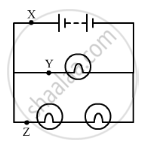Which of the following statements about the currents at X, Y and Z is correct?
(a) The current at Z is greater than that at Y.
(b) The current at Y is greater than that at Z.
(c) The current at X equals the current at Y.
(d) The current at X equals the current at Z.

Exercise 5 | Q 41

V1, V2 and V3 are the p.ds. across the 1Ω, 2Ω and 3Ω resistors in the following diagram, and the current is 5 A.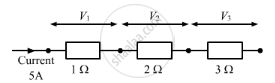Which one of the columns (a) to (d) shows the correct values of V1, V2 and V3measured in volts?

V_1   V_2  V_3

(a)         1.0       2.0        3.0

(b)         5.0       10.0      15.0

(c)         5.0       2.5        1.6

(d)         4.0       4.0        2.0

Exercise 5 | Q 42

A wire of resistance R1 is cut into five equal pieces. These five pieces of wire are then connected in parallel. If the resultant resistance of this combination be R2, then the ratio R_1/R_2 is:

(a) 1/25

(b)1/5

(c)5

(c)25

Exercise 5 | Q 43

Show with the help of diagrams, how you would connect three resistors each of resistance 6 Ω, so that the combination has resistance of (i) 9 Ω (ii) 4 Ω.

Exercise 5 | Q 44

Two resistances when connected in parallel give resultant value of 2 ohm; when connected in series the value becomes 9 ohm. Calculate the value of each resistance.

Exercise 5 | Q 45

A resistor of 8 ohms is connected in parallel with another resistor X. The resultant resistance of the combination is 4.8 ohms. What is the value of the resistor X?

Exercise 5 | Q 46

You are given three resistances of 1, 2 and 3 ohms. Shows by diagrams, how with the help of these resistances you can get:

(i) 6 Ω
(ii) 6/1 Ω
(iii) 1.5 Ω

Exercise 5 | Q 47

How will you connect three resistors of 2 Ω, 3 Ω and 5 Ω respectively so as to obtain a resultant of 2.5 Ω? Draw the diagram to show the arrangement.

Exercise 5 | Q 48

How will you connect three resistors of resistances 2 Ω, 3 Ω, and 6 Ω to obtain a total resistance of  4 Ω, and  1 Ω?

Exercise 5 | Q 49

What is (a) highest, and (b)Ω lowest, resistance which can be obtained by combining four resistors having the following resistances?

4 Ω, 8 Ω, 12 Ω, 24 Ω

Exercise 5 | Q 50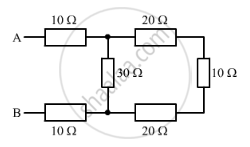Exercise 5 | Q 51

You can given one hundred 1 Ω resistors. What is the smallest and largest resistance you can make in a circuit using these?

Exercise 5 | Q 52

You are supplied with a number of 100 Ω resistors. How could you combine some of these resistors to make a 250 Ω resistor?

Exercise 5 | Q 53

The resistors R1, R2, R3 and R4 in the figure given below are all equal in value.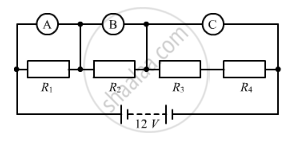What would you expect the voltmeter A, B and C to read assuming that the connecting wires in the circuit have negligible resistance?

Exercise 5 | Q 54

Four resistances of 16 ohms each are connected in parallel. Four such combinations are connected in series. What is the total resistance?

Exercise 5 | Q 55

If the lamps are both the same in the figure given below and if A1 reads 0.50 A, what do A2, A3, A4 and A5 read?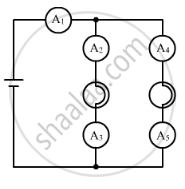Exercise 6

### Lakhmir Singh solutions for Class 10 Physics (Science) Chapter 1 Electricity Exercise 6

Exercise 6 | Q 1

Are the lights in your house wired in series?

Exercise 6 | Q 2

What happens to the other bulbs in a series circuit if one bulb blows off?

Exercise 6 | Q 3

What happens to the other bulbs in a parallel circuit if one bulb blows off?

Exercise 6 | Q 4.1

Which type of circuit, series or parallel, is preferred while connecting a large number of bulbs:

(a) for decorating a hotel building from outside?

Exercise 6 | Q 4.2

Which type of circuit, series or parallel, is preferred while connecting a large number of bulbs:

(b) for lighting inside the rooms of the hotel?

Exercise 6 | Q 5

Draw a circuit diagram to show how two 4 V electric lamps can be lit brightly from two 2 V cells.

Exercise 6 | Q 6

Why is a series arrangement not used for connecting domestic electrical appliances in a circuit?

Exercise 6 | Q 7

Give three reasons why different electrical appliances in a domestic circuit are connected in parallel.

Exercise 6 | Q 8.1

Ten bulbs are connected in a series circuit to a power supply line. Ten identical bulbs are connected in a parallel circuit to an identical power supply line.

(a) Which circuit would have the highest voltage across each bulb?

Exercise 6 | Q 8.2

Ten bulbs are connected in a series circuit to a power supply line. Ten identical bulbs are connected in a parallel circuit to an identical power supply line.

(b) In which circuit, if one bulbs be brighter?

Exercise 6 | Q 8.3

Ten bulbs are connected in a series circuit to a power supply line. Ten identical bulbs are connected in a parallel circuit to an identical power supply line.

(c) In which circuit, if one bulb blows out, all other will stop glowing?

Exercise 6 | Q 8.4

Ten bulbs are connected in a series circuit to a power supply line. Ten identical bulbs are connected in a parallel circuit to an identical power supply line.

(d) Which circuit would have less current in it?

Exercise 6 | Q 9

Consider the circuit given below: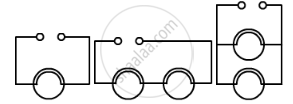(a) In which circuit are the lamps dimmest?
(b) In which circuit or circuits are the lamps of equal brightness to the lamps in circuit (i)?
(c) Which circuit gives out the maximum light?

Exercise 6 | Q 10

If you were going to connect two light bulbs to one battery, would you use a series or a parallel arrangement? Why? Which arrangement takes more current form the batte

Exercise 6 | Q 11.1

Which is the better way to connect lights and other electrical appliances in domestic wiring: series circuits or parallel circuits? why?

Exercise 6 | Q 11.2

Christmas tree lamps are usually wired in series. What happens if one lamps breaks?

Exercise 6 | Q 11.3

An electrician has wired a house in such a way that if a lamp gets fused in one room of the house, all the lamps in other rooms of the house stop working. What is the defect in the wiring?

Exercise 6 | Q 11.4

Draw a circuit diagram showing two electric lamps connected in parallel together with a cell and a switch that works both lamps. Mark an â’¶ on your diagram to show where an ammeter should be placed to measure the current.

Exercise 6 | Q 12

The lamps in a house hold circuit are connected in parallel because:

(a) this way they required less current
(b) if one lamp fails the others remain lit
(c) this way they require less power
(d) if one lamp fails the other also fail

Exercise 6 | Q 13

Using the circuit given below, state which of the following statement is correct?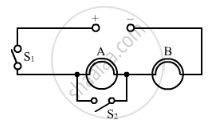(a) When S1 and S2 are closed, lamps A and B are lit.22
(b) With S1 open and S2 closed, A is lit and B is not lit.
(c) With S2 open and S1 closed A and B are lit.
(d) With S1 closed and S2 open, lamp A remains lit even if lamp B gets fused.

Exercise 6 | Q 14

Draw a circuit diagram showing two lamps, one cell and a switch connected in series.
How can you change the brightness of the lamp?

Exercise 6 | Q 15

Consider the circuit given below when A, B and C are three identical light bulbs of constant resistance.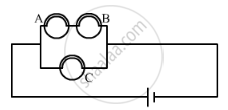(a) List the bulbs in order of increasing brightness.
(b) If C burns out, what will be the brightness of A now compared with before?
(c) If B burns out instead, what will be the brightness of A and C compared with before?

Exercise 6 | Q 16

How do you think the brightness of two lamps arranged in parallel compares with the brightness of two lamps arranged in series (both arrangements having one cell)?

Exercise 6 | Q 17

If current flows through two lamps arranged:

(a) in series,
(b) in parallel,
and the filament of one lamps breaks, what happens to the other lamp? Explain your answer.

Exercise 6 | Q 18

The figure blow shows a variable resistor in a dimmer switch.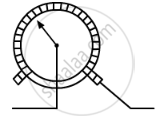How would you turn the switch to make the lights: (a) brighter, and  (b) dimmer? Explain your answer.

Exercise 7

### Lakhmir Singh solutions for Class 10 Physics (Science) Chapter 1 Electricity Exercise 7

Exercise 7 | Q 1

State two factors on which the electrical energy consumed by an electrical appliance depends.

Exercise 7 | Q 2

Which one has a higher electrical resistance : a 100 watt bulb or a 60 watt bulb?

Exercise 7 | Q 3

Name the commercial unit of electric energy.

Exercise 7 | Q 4

An electric bulb is rated at 220 V, 100 W. What is its resistance?

Exercise 7 | Q 5

What is the SI unit of (i) electric energy, and (ii) electric power?

Exercise 7 | Q 6

Name the quantity whose unit is (i) kilowatt, and (ii) kilowatt-hour.

Exercise 7 | Q 7

Which quantity has the unit of watt?

Exercise 7 | Q 8

What is the meaning of the symbol kWh? Which quantity does it represent?

Exercise 7 | Q 9

If the potential difference between the end of a wire of fixed resistance is doubled, by how much does the electric power increase?

Exercise 7 | Q 10

An electric lamp is labelled 12 V, 36 W. This indicates that it should be used with a 1 V supply. What other information does the label provide?

Exercise 7 | Q 11

What current will be taken by a 920 W appliance if the supply voltage is 230 V?

Exercise 7 | Q 12

Define watt. Write down an equation linking watts, volts and amperes.

Exercise 7 | Q 13

Define watt-hour. How many joules are equal to 1 watt-hour?

Exercise 7 | Q 14

How much energy is consumed when a current of 5 amperes flows through the filament (or element) of a heater having resistance of 100 ohms for two hours? Express it in joules.

Exercise 7 | Q 15

An electric bulb is connected to a 220 V power supply line. If the bulb draws a current of 0.5 A, calculate the power of the bulb.

Exercise 7 | Q 16

In which of the following cases more electrical energy is consumed per hour?

(i) A current of 1 ampere passed through a resistance of 300 ohms.
(ii) A current of 2 amperes passed through a resistance of 100 ohms.

Exercise 7 | Q 17

An electric kettle rated at 220 V, 2.2 kW, works for 3 hours. Find the energy consumed and the current drawn.

Exercise 7 | Q 18

In a house two 60 W electric bulbs are lighted for 4 hours, and three 100 W bulbs for 5 hours everyday. Calculate the electric consumed in 30 days.

Exercise 7 | Q 19

A bulb is rated as 250 V; 0.4 A. Find its : (i) power, and (ii) resistance.

Exercise 7 | Q 20

For a heater rated at 4 kW and 220 V, calculate:

(a) the current,
(b) the resistance of the heater,
(c) the energy consumed in 2 hours, and
(d) the cost if 1 kWh is priced at Rs 4.60.

Exercise 7 | Q 21

An electric motor takes 5 amperes current form a 220 volt supply line. Calculate the power of the motor and electric energy consumed by it in 2 hours.

Exercise 7 | Q 22

Which uses more energy : a 250 W TV set in 1 hour or a 1200 W toaster in 10 minutes?

Exercise 7 | Q 23.1

Calculate the power used in the 2 Ω resistor in each of the following circuits: a 6 V battery in series with 1 Ω and 2 Ω resistors.

Exercise 7 | Q 23.2

Calculate the power used in the 2 Ω resistor in each of the following circuits: a 4 V battery in parallel with 12 Ω and 2 Ω resistors.

Exercise 7 | Q 24

Two lamps, one rated 40 W at 220 V and the other 60 W at 220 V, are connected in parallel to the electric supply at 220 V.

(a) Draw a circuit diagram to show the connections.
(b) Calculate the current drawn from the electric supply.
(c) Calculate the total energy consumed by the two lamps together when they operate for one hour.

Exercise 7 | Q 25

An electric kettle connected to the 230 V mains supply draws a current of 10 A. Calculate:

(a) the power of the kettle.
(b) the energy transferred in 1 minute.

Exercise 7 | Q 26

An electric fan runs from the 230 V mains. The current flowing through it is 0.4 A. At what rate is electrical energy transferred by the fan?

Exercise 7 | Q 26

A 2 kWh heater, a 200 W TV and three 100 W lamps are all switched on from 6 p.m. to 10 p.m. What is the total cost at Rs 5.50 per kWh?

Exercise 7 | Q 27

What is the maximum power in kilowatts of the appliance that can be connected safely to a 13 A ; 230 V mains socket?

Exercise 7 | Q 29.1

What is meant by " electric power"

Exercise 7 | Q 29.1

Write the formula for electric power in terms of potential difference and current.

Exercise 7 | Q 29.1

Write the formula for electric power in terms of potential difference and current.

Exercise 7 | Q 29.2

The diagram below shows a circuit containing a lamp L, a voltmeter and an ammeter,. The voltmeter reading 3 V and the ammeter reading is 0.5 A.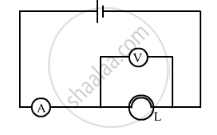What is the resistance of the lamp?
What is the power of the lamp?

Exercise 7 | Q 29.3

Define kilowatt-hour.

Exercise 7 | Q 29.3

How many joules are there in one kilowatt-hour?

Exercise 7 | Q 29.4

Calculate the cost of operating a heater of 500 W for 20 hours at the rate of Rs 3.90 per unit.

Exercise 7 | Q 30

When an electric lamp is connected to 12 V battery, it draws a current of 0.5 A. The power of the lamp is:

(a) 0.5 W
(b) 6 W
(c) 12 W
(d) 24 W

Exercise 7 | Q 31

The unit for expressing electric power is:

(a) volt
(b) joule
(c) coulomb
(d) watt

Exercise 7 | Q 32

Which of the following is likely to be the correct wattage for an electric iron used in our homes?

(a) 60 W
(b) 250 W
(c) 850 W
(d) 2000 W

Exercise 7 | Q 33

An electric heater is rated at 2 kW. Electrical energy costs Rs 4 per kWh. What is the cost of using the heater for 3 hours?

(a) Rs 12
(b) Rs 24
(c) Rs 36
(d) Rs 48

Exercise 7 | Q 34

The SI unit of energy is :

(a) joule
(b) coulomb
(c) watt
(d) ohm-metre

Exercise 7 | Q 35

The commercial unit of energy is :

(a) watt
(b) watt-hour
(c) kilowatt-hour
(d) kilo-joule

Exercise 7 | Q 36

How much energy does a 100 W electric bulb transfer in 1 minute?

(a) 100 J
(b) 600 J
(c) 3600 J
(d) 6000 J

Exercise 7 | Q 37

An electric kettle for use on a 230 V supply is rated 3000 W. For safe working, the cable connected to it should be able to carry at least:

(a) 2 A
(b) 5 A
(c) 10 A
(d) 15 A

Exercise 7 | Q 38

How many joules of electrical energy are transferred per second by a 6 V; 0.5 A lamp?

(a) 30 J/s
(b) 12 J/s
(c) 0.83 J/s
(d) 3 J/s

Exercise 7 | Q 38

How many joules of electrical energy are transferred per second by a 6 V; 0.5 A lamp?

(a) 30 J/s
(b) 12 J/s
(c) 0.83 J/s
(d) 3 J/s

Exercise 7 | Q 38

How many joules of electrical energy are transferred per second by a 6 V; 0.5 A lamp?

(a) 30 J/s
(b) 12 J/s
(c) 0.83 J/s
(d) 3 J/s

Exercise 7 | Q 38

How many joules of electrical energy are transferred per second by a 6 V; 0.5 A lamp?

(a) 30 J/s
(b) 12 J/s
(c) 0.83 J/s
(d) 3 J/s

Exercise 7 | Q 38

How many joules of electrical energy are transferred per second by a 6 V; 0.5 A lamp?

(a) 30 J/s
(b) 12 J/s
(c) 0.83 J/s
(d) 3 J/s

Exercise 7 | Q 38

How many joules of electrical energy are transferred per second by a 6 V; 0.5 A lamp?

(a) 30 J/s
(b) 12 J/s
(c) 0.83 J/s
(d) 3 J/s

Exercise 7 | Q 38

How many joules of electrical energy are transferred per second by a 6 V; 0.5 A lamp?

(a) 30 J/s
(b) 12 J/s
(c) 0.83 J/s
(d) 3 J/s

Exercise 7 | Q 38

How many joules of electrical energy are transferred per second by a 6 V; 0.5 A lamp?

(a) 30 J/s
(b) 12 J/s
(c) 0.83 J/s
(d) 3 J/s

Exercise 7 | Q 38

How many joules of electrical energy are transferred per second by a 6 V; 0.5 A lamp?

(a) 30 J/s
(b) 12 J/s
(c) 0.83 J/s
(d) 3 J/s

Exercise 7 | Q 39

At a given time, a house is supplied with 100 A at 220 V. How many 75 W, 220 V light bulb could be switched on in the house at the same time (if they are all connected in parallel)?

• 93

• 193

• 293

• 393

Exercise 7 | Q 40

If the potential difference between the ends of a fixed resistor is halved, the electric power will become:

• double

• half

• four times

• one-fourth

Exercise 7 | Q 41

State whether an electric heater will consume more electrical energy or less electrical energy per second when the length of its heating element is reduced. Give reasons for your answer.

Exercise 7 | Q 42

The table below shows the current in three different electrical appliances when connected to the 240 V mains supply:

Appliance            Current

Kettle                 08.5A

Lamp                  0.4A

toaster                 4.8A

(a) Which appliance has the greatest electrical resistance? How does the data show this?
(b) The lamp is connected to the mains supply by using a thin, twin-cored cable consisting of live and natural wires. State two reasons why this cable should not be used for connecting the kettle to the mains supply.
(c) Calculate the power rating of the kettle when it is operated from the 240 V mains supply.
(d) A main takes the kettle abroad where the mains supply is 120 V. What is the current is the kettle when it is operated from the 120 V supply?

Exercise 7 | Q 43

A boy noted the reading on his home's electricity meter on Sunday at 8 AM and again on Monday at 8 AM (see Figures below).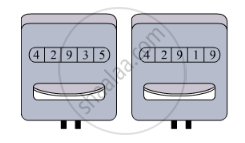(a) What was the meter reading on Sunday?
(b) What was the meter reading on Monday?
(c) How many units of electricity have been used?
(d) In how much time these units have been used?
(e) If the rate is Rs 5 per unit, what is the cost of electricity used during this time?

Exercise 7 | Q 44

An electric bulb is rated as 10 W, 220 V. How many of these bulbs can be connected in parallel across the two wires of 220 V supply line if the maximum current which can be drawn is 5 A?

Exercise 7 | Q 45

Two exactly similar electric lamps are arranged (i) in parallel, and (ii) in series. If the parallel and series combination of lamps are connected to 220 V supply line one by one, what will be the ratio of electric power consumed by them?

Exercise 8

### Lakhmir Singh solutions for Class 10 Physics (Science) Chapter 1 Electricity Exercise 8

Exercise 8 | Q 1

How does the heat H produced by a current passing through a fixed resistance wire depend on magnitude of current I?

Exercise 8 | Q 2

If the current passing through a conductor is doubled, what will be the change in heat produced

Exercise 8 | Q 3

Name two effects produced by electric current.

Exercise 8 | Q 4

Which effect of current is utilised in an electric light bulb?

Exercise 8 | Q 5

Which effect of current is utilised in the working of an electric fuse?

Exercise 8 | Q 6

Name two devices which work on the heating effect of electric current.

Exercise 8 | Q 7

Name two gases which are filled in filament type electric light bulbs.

Exercise 8 | Q 8

Explain why, filament type electric bulbs are not power efficient.

Exercise 8 | Q 9

Why does the connecting cord of an electric heater not glow hot while the heating element does?

Exercise 8 | Q 10.1

Write down the formula for the heat produced when a current I is passed through a resistor R for time t.

Exercise 8 | Q 10.2

An electric iron of resistance 20 ohms draws a current of 5 amperes. Calculate the heat produced in 30 seconds.

Exercise 8 | Q 11

State three factors on which the heat produced by an electric current depends. How does it depend on these factors?

Exercise 8 | Q 12.1

Write Joule's law of heating.

Exercise 8 | Q 12.2

A resistance of 40 ohms and one of 60 ohms are arranged in series across 220 volt supply. Find the heat in joules produced by this combination of resistances in half a minute.

Exercise 8 | Q 13

Why is an electric light bulb not filled with air? Explain why argon or nitrogen is filled in an electric bulb.

Exercise 8 | Q 14

Explain why, tungsten is used for making the filaments of electric bulbs.

Exercise 8 | Q 15

Explain why, the current that makes the heater element very hot, only slightly warms the connecting wires leading to the heater.

Exercise 8 | Q 16

When a current of 4.0 A passes through a certain resistor for 10 minutes, 2.88 × 10J of heat are produced. Calculate

the power of the resistor.
the voltage across the resistor.

Exercise 8 | Q 17

A heating coil has a resistance of 200 Ω. At what rate will heat be produced in it when a current of 2.5 A flows through it?

Exercise 8 | Q 18

An electric heater of resistance 8 Ω takes a current of 15 A from the mains supply line. Calculate the rate at which heat is developed in the heater.

Exercise 8 | Q 19

A resistance of 25 Ω is connected to a 12 V battery. Calculate the heat energy in joules generated per minute.

Exercise 8 | Q 20

100 joules of heat is produced per second in a 4 ohm resistor. What is the potential difference across the resistor?

Exercise 8 | Q 21.1

Derive the expression for the heat produced due to a current 'I' flowing for a time interval 't' through a resistor 'R' having a potential difference 'V' across its ends. With which name is this relation known?

Exercise 8 | Q 21.2

How much heat will an instrument of 12 W produce in one minute if it is connected to the heat produced by it?

Exercise 8 | Q 21.3

The current passing through a room heater has been halved. What will happen to the heat produced by it?

Exercise 8 | Q 21.4

What is meant by the heating effect of current? Give two applications of the heating effect of current.

Exercise 8 | Q 21.5

Name the material which is used for making the filaments of an electric bulb.

Exercise 8 | Q 22

The heat produced by passing an electric current through a fixed resistor is proportional to the square of:

(a) magnitude of resistance of the resistor
(b) temperature of the resistor
(c) magnitude of current
(d) time for which current is passed

Exercise 8 | Q 23

The current passing through an electric kettle has been doubled. The heat produced will become:

(a) half
(b) double
(c) four time
(d) one-fourth

Exercise 8 | Q 24

An electric fuse works on the:
(a) chemical effect of current
(b) magnetic effect of current
(c) lighting effect of current
(d) heating effect of current

Exercise 8 | Q 25

The elements of electrical heating devices are usually made of:

(a) tungsten
(b) bronze
(c) nichrome
(d) argon

Exercise 8 | Q 26

The heat produced in a wire of resistance 'x' when a current 'y' flows through it in time 'z' is given by:

(a) x2 × y × z
(bx × z × y2
(cy × z2 × x
(dy × z ×

Exercise 8 | Q 27

Which of the following characteristic is not suitable for a fuse wire?

(a) thin and short
(b) thick and short
(c) low melting point
(d) higher resistance than rest of wiring

Exercise 8 | Q 28

In a filament type light bulb, most of the electric power consumed appears as:

(a) visible light
(b) infra-red-rays
(c) ultraviolet rays
(d) fluorescent light

Exercise 8 | Q 29

Which of the following is the most likely temperature of the filament of an electric light bulb when it is working on the normal 220 V supply line?

(a) 500°C
(b) 1500°C
(c) 2500°C
(d) 4500°C

Exercise 8 | Q 30

If the current flowing through a fixed resistor is halved, the heat produced in it will become:

• double

• one-half

• one-fourth

• four times

Exercise 8 | Q 31

The electrical resistivities of four materials P, Q, R and S are given below:

 P 6.84xx10^8Ωm Q 1.70xx10^8Ωm R  1.0xx10^15Ωm s 11.0xx10^-7Ωm

Which material will you use for making: (a) heating element of electric iron (b) connecting wires of electric iron (c) covering of connecting wires? Give reason for your choice in each case.

Exercise 8 | Q 32

How does the wire in the filament of a light bulb behave differently to the other wires in the circuit when the current flows?

What property of the filament wire accounts for this difference?

Exercise 8 | Q 33

Two exactly similar heating resistances are connected (i) in series, and (ii) in parallel, in two different circuits, one by one. If the same current is passed through both the combinations, is more heat obtained per minute when they are connected in series or when they are connected in parallel? Give reason for your answer.

Exercise 8 | Q 35

Which electric heating device in your home do you think have resistors which control the flow of electricity?

## Solutions for Chapter 1: Electricity

Exercise 1Exercise 2Exercise 3Exercise 4Exercise 5Exercise 6Exercise 7Exercise 8## Lakhmir Singh solutions for Class 10 Physics (Science) chapter 1 - Electricity

Shaalaa.com has the CBSE Mathematics Class 10 Physics (Science) CBSE solutions in a manner that help students grasp basic concepts better and faster. The detailed, step-by-step solutions will help you understand the concepts better and clarify any confusion. Lakhmir Singh solutions for Mathematics Class 10 Physics (Science) CBSE 1 (Electricity) include all questions with answers and detailed explanations. This will clear students' doubts about questions and improve their application skills while preparing for board exams.

Further, we at Shaalaa.com provide such solutions so students can prepare for written exams. Lakhmir Singh textbook solutions can be a core help for self-study and provide excellent self-help guidance for students.

Concepts covered in Class 10 Physics (Science) chapter 1 Electricity are Electricity, Electric Current, Ohm's Law (V = IR), Electrical Power, Electric Circuit, Factors Affecting the Resistance of a Conductor, Resistances in Parallel, Effects of Electric Current, Heating Effect of Electric Current, Resistors in Series, Potential and Potential Difference, Symbols and Functions of Various Components of an Electric Circuits, Electrical Resistivity and Electrical Conductivity.

Using Lakhmir Singh Class 10 Physics (Science) solutions Electricity exercise by students is an easy way to prepare for the exams, as they involve solutions arranged chapter-wise and also page-wise. The questions involved in Lakhmir Singh Solutions are essential questions that can be asked in the final exam. Maximum CBSE Class 10 Physics (Science) students prefer Lakhmir Singh Textbook Solutions to score more in exams.

Get the free view of Chapter 1, Electricity Class 10 Physics (Science) additional questions for Mathematics Class 10 Physics (Science) CBSE, and you can use Shaalaa.com to keep it handy for your exam preparation.